C Program to find Largest Element in Matrix

In this tutorial you will learn about the C Program to find Largest Element in Matrix and its application with practical example.

C Program to Find Largest Element in Matrix

In this tutorial, we will learn to create a C program that will find the Largest Element in Matrix using C programming.

Prerequisites

Before starting with the tutorial we assume that you are best aware of the following C programming topics:

• Operators in C Programming.
• Basic Input and Output function in C Programming.
• Basic C programming.
• For loop in C programming.
• Conditional statement in C programming.

Program to find Largest Element in Matrix

In c programming, it is possible to take a numerical input for the size of the matrix and the elements of the matrix from the user and find the Largest Element in Matrix with the help of a very small amount of code. The C language has many types of header libraries which has supported function in them with the help of these files the programming is easy.

Algorithm:-

With the help of this program, we can find the Largest Element in Matrix.

Output:-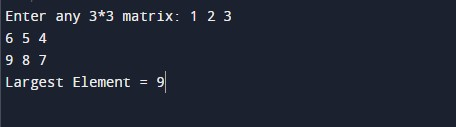In the above program, we have first initialized the required variable.

• mat= it will hold the integer value.
• i= it will hold the integer value.
• j= it will hold the integer value.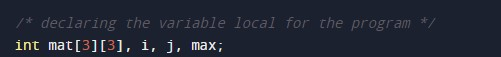Taking the elements of the matrix from the user.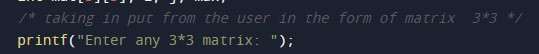Calculating the largest element matrix.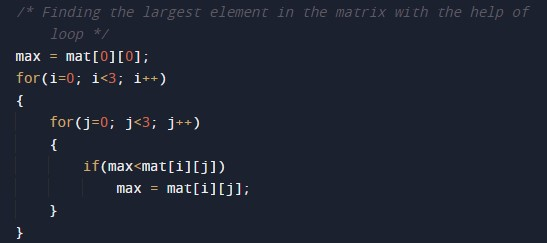Printing the largest element of the matrix.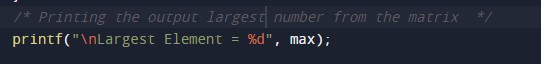In this tutorial we have learn about the C Program to find Largest Element in Matrix and its application with practical example. I hope you will like this tutorial.